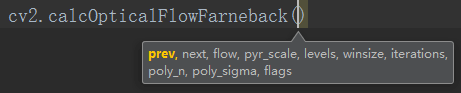# 基于Python的OpenCV图像处理17

## Jun 28,2017   10204 words   37 min

#### 一、Shi-Tomasi角点检测算子

##### 1.原理

Shi-Tomasi角点检测算子的原理很简单，而且主要思想与Harris算子类似。不同之处在于打分函数R。 在Harris算子中，打分函数如下：

$R=det(M)-k(trace(M))^{2}$

$R=min(\lambda _{1},\lambda _{2})$

##### 2.OpenCV实现

# coding=utf-8
import cv2
import numpy as np

gray = cv2.cvtColor(img, cv2.COLOR_BGR2GRAY)

# 第一个参数是输入图像
# 第二个参数是检测的角点数目
# 第三个参数是角点质量
# 第四个参数是角点间的最短距离
corners = cv2.goodFeaturesToTrack(gray, 300, 0.01, 10)

# 将浮点型数据转成int型，否则无法绘制
corners = np.int0(corners)

for i in corners:
# 将每一项拆开，在重新拼成一个元组
x, y = i.ravel()
# 在图上绘制角点，为了更好显示，设置半径为2
cv2.circle(img, (x, y), 2, (0, 0, 255), -1)

cv2.imshow("shi-tomasi", img)
cv2.waitKey(0)


#### 二、光流法视频目标跟踪

##### 1.光流的概念

• 1.在连续的两帧图像之间（目标对象的）像素的灰度值不改变。
• 2.相邻的像素具有相同的运动。
##### 2.光流方程推导

$I(x,y,t)=I(x+dx,y+dy,t+dt)$

$\frac{\partial I}{\partial x}\frac{\Delta x}{\Delta t}+\frac{\partial I}{\partial y}\frac{\Delta y}{\Delta t}+\frac{\partial I}{\partial t}\frac{\Delta t}{\Delta t}=0$

$V_{x}=\frac{\Delta x}{\Delta t}$ $V_{y}=\frac{\Delta y}{\Delta t}$

$\frac{\partial I}{\partial x}V_{x}+\frac{\partial I}{\partial y}V_{y}+\frac{\partial I}{\partial t}=0$

$I_{x}=\frac{\partial I}{\partial x},I_{y}=\frac{\partial I}{\partial y},I_{t}=\frac{\partial I}{\partial t}$

$I_{x}V_{x}+I_{y}V_{y}+I_{t}=0$

$I_{x}(p_{1})V_{x}+I_{y}(p_{1})V_{y}=-I_{t}(p_{1}) \\I_{x}(p_{2})V_{x}+I_{y}(p_{2})V_{y}=-I_{t}(p_{2}) \\\vdots \\I_{x}(p_{n})V_{x}+I_{y}(p_{n})V_{y}=-I_{t}(p_{n})$

$A=\begin{bmatrix} I_{x}(p_{1}) & I_{y}(p_{1})\\ I_{x}(p_{2}) & I_{y}(p_{2})\\ \vdots & \vdots\\ I_{x}(p_{n}) & I_{y}(p_{n}) \end{bmatrix} , x=\begin{bmatrix} V_{x}\\ V_{y} \end{bmatrix} ,b=\begin{bmatrix} -I_{t}(p_{1})\\ -I_{t}(p_{2})\\ \vdots \\ -I_{t}(p_{n}) \end{bmatrix}$

$Ax=b\\ A^{T}Ax=A^{T}b\\ x=(A^{T}A)^{-1}A^{T}b$

$A^{T}A=\begin{bmatrix} I_{x}(p_{1}) &I_{x}(p_{2}) & \cdots &I_{x}(p_{n}) \\ I_{y}(p_{1}) &I_{y}(p_{2}) & \cdots &I_{y}(p_{n}) \end{bmatrix}\begin{bmatrix} I_{x}(p_{1}) & I_{y}(p_{1})\\ I_{x}(p_{2}) & I_{y}(p_{2})\\ \vdots & \vdots\\ I_{x}(p_{n}) & I_{y}(p_{n}) \end{bmatrix}\\ =\begin{bmatrix} \sum_{i=1}^{n}I_{x}(p_{i}) & \sum_{i=1}^{n}I_{x}(p_{i})I_{y}(p_{i})\\ \sum_{i=1}^{n}I_{y}(p_{i})I_{x}(p_{i}) & \sum_{i=1}^{n}I_{y}(p_{i}) \end{bmatrix}$

A转置乘以b如下：

$A^{T}b=\begin{bmatrix} I_{x}(p_{1}) &I_{x}(p_{2}) & \cdots &I_{x}(p_{n}) \\ I_{y}(p_{1}) &I_{y}(p_{2}) & \cdots &I_{y}(p_{n}) \end{bmatrix}\begin{bmatrix} -I_{t}(p_{1})\\ -I_{t}(p_{2})\\ \vdots \\ -I_{t}(p_{n}) \end{bmatrix}\\ =\begin{bmatrix} -\sum_{i=1}^{n}I_{x}(p_{i})I_{t}(p_{i})\\ -\sum_{i=1}^{n}I_{y}(p_{i})I_{t}(p_{i}) \end{bmatrix}$

$x=\begin{bmatrix} V_{x}\\ V_{y} \end{bmatrix}\\ =\begin{bmatrix} \sum_{i=1}^{n}I_{x}(p_{i}) & \sum_{i=1}^{n}I_{x}(p_{i})I_{y}(p_{i})\\ \sum_{i=1}^{n}I_{y}(p_{i})I_{x}(p_{i}) & \sum_{i=1}^{n}I_{y}(p_{i}) \end{bmatrix}^{-1}\begin{bmatrix} -\sum_{i=1}^{n}I_{x}(p_{i})I_{t}(p_{i})\\ -\sum_{i=1}^{n}I_{y}(p_{i})I_{t}(p_{i}) \end{bmatrix}$

# coding=utf-8
import numpy as np
import cv2

cap = cv2.VideoCapture("E:\\v1.mp4")

# Shi-Tomasi角点检测相关参数
feature_params = dict(maxCorners=10,
qualityLevel=0.1,
minDistance=1,
blockSize=9)

# LK光流法相关参数
lk_params = dict(winSize=(30, 30),
maxLevel=2,
criteria=(cv2.TERM_CRITERIA_EPS | cv2.TERM_CRITERIA_COUNT, 10, 0.03))

# 创建随机颜色
color = np.random.randint(0, 255, (100, 3))

# 获取视频第一帧
# 转换为灰度
old_gray = cv2.cvtColor(old_frame, cv2.COLOR_BGR2GRAY)
# ST检测角点，注意这里参数传递的方法

# 创建一个新的图片用于绘制

while 1:

if frame is None:
cv2.waitKey(0)
break
else:
frame_gray = cv2.cvtColor(frame, cv2.COLOR_BGR2GRAY)
# 计算光流
p1, st, err = cv2.calcOpticalFlowPyrLK(old_gray, frame_gray, p0, None, **lk_params)

# 选择好的特征点
if p1 is None:
pass
elif p0 is None:
pass
else:
good_new = p1[st == 1]
good_old = p0[st == 1]

# 输出每一帧内特征点的坐标
# 坐标个数为之前指定的个数
print good_new

# 绘制轨迹
for i, (new, old) in enumerate(zip(good_new, good_old)):
a, b = new.ravel()
c, d = old.ravel()
frame = cv2.circle(frame, (a, b), 5, color[i].tolist(), -1)

cv2.imshow('frame', img)
k = cv2.waitKey(30) & 0xff
if k == 27:
break

# 更新上一帧以及特征点
old_gray = frame_gray.copy()
p0 = good_new.reshape(-1, 1, 2)

cv2.destroyAllWindows()
cap.release()


##### 5.稠密光流

calcOpticalFlowFarneback(prevImg, nextImg, flow, pyr_scale, levels, winsize, iterations, poly_n, poly_sigma, flags[, flow])• prevImg:输入的8bit单通道前一帧图像；
• nextImg:输入的8bit单通道当前帧图像；
• flow:光流计算结果会保存在这个参数指定的变量中，可以设置为None，然后用赋值方式赋给其它变量；
• pyr_scale:金字塔参数(<1)，0.5为经典参数，表示每一层是下一层尺度的一半；
• levels:金字塔的层数；
• winsize:窗口大小；
• iterations:迭代次数；
• poly_n:像素邻域的大小，一般为5或7，大的话表示图像整体比较平滑。size of the pixel neighborhood used to find polynomial expansion in each pixel;typically poly_n =5 or 7.
• poly_sigma:高斯标准差。standard deviation of the Gaussian that is used to smooth derivatives used as a basis for the polynomial expansion; for poly_n=5, you can set poly_sigma=1.1, for poly_n=7, a good value would be poly_sigma=1.5.
• flags:可以为下列的组合：cv2.OPTFLOW_USE_INITIAL_FLOW(0)和cv2.OPTFLOW_FARNEBACK_GAUSSIAN(1)；0计算快，1慢但准确。

• Horn-Schunck法 cv2.calcOpticalFlowHS()
• 块匹配法 cv2.calcOpticalFlowBM()

# coding=utf-8
import cv2
import numpy as np

cap = cv2.VideoCapture("E:\\vtest.avi")

prvs = cv2.cvtColor(frame1, cv2.COLOR_BGR2GRAY)

# 注意这里新建一个矩阵的用法
# 等价于hsv = np.zeros(frame1.shape,np.uint8)
# 但这样更加简单
hsv = np.zeros_like(frame1)

# 注意这里的用法，等价于hsv[:,:,1]=255
# 目的是把HSV的S通道全部赋成255
# S表示饱和度，即让饱和度为100%
# 这里还有个问题，为什么用HSV色彩空间
# 因为我们想用不同颜色表示不同方向，不同明暗表示大小
# 因此HSV空间最适合，最方便满足这个要求
# 如果RGB空间则很难直接办到了
# HSV面向用户，RGB面向计算机
# 从用户角度而言，HSV空间更接近于我们对于色彩的理解
hsv[..., 1] = 255

while 1:
next = cv2.cvtColor(frame2, cv2.COLOR_BGR2GRAY)

# 使用Gunnar Farneback方法进行稠密光流计算
# 第一个参数是上一帧影像
# 第二个参数是下一帧影像
# 第三个参数是接收结果的变量，可为空
# 第四个参数是金字塔缩放参数，每层为上层大小的0.5
# 第五个参数是金字塔层数
# 第六个参数是窗口大小
# 第七个参数是迭代次数
# 第八个参数是像素领域大小
# 第九个参数是高斯标准差
# 第十个参数是flag，表示计算方式
flow = cv2.calcOpticalFlowFarneback(prvs, next, None, 0.5, 3, 15, 3, 5, 1.2, cv2.OPTFLOW_USE_INITIAL_FLOW)

# cartToPolar函数用于计算一个二维向量的大小和角度
# flow[...,0]等价于flow[:,:,0]
# 返回的mag和ang分别是二维的一层矩阵，存放对应位置的信息，如(576, 768)
mag, ang = cv2.cartToPolar(flow[..., 0], flow[..., 1])

# 将弧度转换为角度，同时OpenCV中的H范围是180(0 - 179)，所以再除以2
# 完成后将结果赋给HSV的H通道，不同的角度(方向)以不同颜色表示
# 对于不同方向，产生不同色调
# hsv[...,0]等价于hsv[:,:,0]
hsv[..., 0] = ang * 180 / np.pi / 2

# 将矢量大小标准化到0-255范围。因为OpenCV中V分量对应的取值范围是256
# 对于同一H、S而言，向量的大小越大，对应颜色越亮
hsv[..., 2] = cv2.normalize(mag, None, 0, 255, cv2.NORM_MINMAX)
# 最后，将生成好的HSV图像转换为BGR颜色空间
rgb = cv2.cvtColor(hsv, cv2.COLOR_HSV2BGR)
cv2.imshow('frame2', rgb)

# 退出控制
k = cv2.waitKey(30) & 0xff
if k == 27:
break
# 按s保存当前帧以及光流图
elif k == ord('s'):
cv2.imwrite('E:\\opticalfb.png', frame2)
cv2.imwrite('E:\\opticalhsv.png', rgb)
prvs = next

cap.release()
cv2.destroyAllWindows()


#### 三、HSV色彩空间

import cv2
import numpy as np

def nothing(x):
pass

img = np.zeros((200, 500, 3), np.uint8)
rgb = np.zeros_like(img)

cv2.namedWindow("HSV")
cv2.createTrackbar('H', 'HSV', 0, 179, nothing)
cv2.createTrackbar('S', 'HSV', 0, 255, nothing)
cv2.createTrackbar('V', 'HSV', 0, 255, nothing)

while 1:
cv2.imshow("HSV", rgb)

h = cv2.getTrackbarPos('H', 'HSV')
s = cv2.getTrackbarPos('S', 'HSV')
v = cv2.getTrackbarPos('V', 'HSV')

img[..., 0] = h
img[..., 1] = s
img[..., 2] = v

rgb = cv2.cvtColor(img, cv2.COLOR_HSV2BGR)

k = cv2.waitKey(1) & 0xff
if k == 27:
break

cv2.waitKey(0)